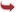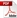International Journal of Scientific and Research Publications

#### IJSRP, Volume 4, Issue 7, July 2014 Edition [ISSN 2250-3153]Determining the relationship between the dielectric properties and the basic physical and chemical parameters of the air-dry soil
L. K. Dospatliev, I. T. Ivanov, B. K. Paarvanova, N. T. Katrandzhiev, R. T. Popova
Abstract: Measurements of the electric conductivity, σ, and relative dielectric permittivity, εr, were conducted (0.1Hz –15 MHz) on 40 air-dried soil samples that were subsequently analyzed for pH, total organic matter in soil (ΔM/m1), P2O5, , Fe2O3 and heavy metal concentrations (Pb, Cd, Cr, Ni, Cu и Zn). The pH of soil samples varied between pH 5.25 and pH 7.73 (mean pH 6.71), the ΔM/m1 varied between 1,49 % and 9.96 % (mean 4.56 %). The mean content of Fe2O3 was 44352.5 mg/kg, which was 114 fold higher than the mean concentration of the heavy metals (Pb, Cd, Cr, Ni, Cu и Zn). The mean content of P2O5 was 0.26% . We found a linear relation between σ (1 MHz) and the indicated physicochemical parameters; P2O5 (coefficient of correlation, r = 0.637), рН (R = 0.530 ) , Fe2O3 concentration (r = 0.450), content of ΔM/m1 (r = 0.545) and heavy metal concentration (r = 0.460). Similar relationships and correlation coefficients were found between εr (10 kHz) and the same physicochemical parameters.
[VIEW FULL PAPER][DOWNLOAD]

Reference this Research Paper (copy & paste below code):

L. K. Dospatliev, I. T. Ivanov, B. K. Paarvanova, N. T. Katrandzhiev, R. T. Popova (2018); Determining the relationship between the dielectric properties and the basic physical and chemical parameters of the air-dry soil; Int J Sci Res Publ 4(7) (ISSN: 2250-3153). http://www.ijsrp.org/research-paper-0714.php?rp=P312859